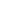# Blekinge Tekniske HogskoleDifferential Equations and Their Symmetries Via Mathematical Models is a Course

## Differential Equations and Their Symmetries Via Mathematical Models

Mar 11 - Apr 29, 2019

### Full course description

##### Course Date:

Mar 11 - Apr 29, 2019

4 weeks

3 hrs/week

Calculus

Self-paced

Certificate

### Description

As a participant in this course, you’ll be introduced to mathematical models formulated in terms of ordinary and partial differential equations. It has been shown that symmetries help us to better understand methods of integration of differential equations and to find new classes of integrable equations. The course exposition includes the Malthusian model of population growth, the comparison of a free fall with the fall of a body in Earth’s atmosphere, an explanation of the collapse of driving shafts in motor ships, the mathematical model of tumor growth, the symmetry nature of wave beams in the deep ocean, and more.

### Objectives

• Get an overview of some mathematical models formulated by differential equations and approaches to solving them.
• Comprehend the difference between modelling by differential equations and by functions.
• Understand the concept of symmetry of a differential equation.
• Use symmetry to solve differential equations and to find new solvable mathematical models.

Prerequisite: Calculus and some acquaintance with differential equations.

Course is offered by Blekinge Tekniske Hogskole.

### Course Instructors#### Dr. Nail Ibragimov

Instructor

Dr. Nail Ibragimov is a professor at the Department of Mathematics and Science, Blekinge Institute of Technology in Sweden. He is widely regarded as one of the world's foremost experts in the field of differential equations, group analysis, and mathematical modelling.#### Elena Avdonina

Instructor

Elena Avdonina is a senior researcher at Ufa State Aviation Technical University (Russia) and an invited researcher at the Department of Mathematics and Natural Sciences, Blekinge Institute of Technology (Sweden).#### Aliia Gainetdinova

Lecturer

Aliia Gainetdinova is a senior lecturer at the Department of high-performance computing technologies and systems at Ufa State Aviation Technical University (Ufa, Russia).